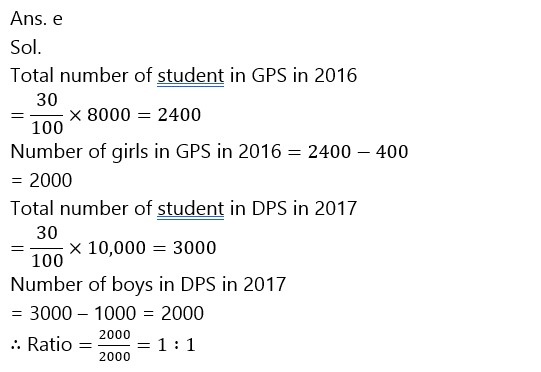# SBI PO Mains Quantitative Aptitude Quiz- 4

## SBI PO Mains Quantitative Aptitude Quiz

Quantitative aptitude measures a candidate’s numerical proficiency and problem-solving abilities. It is the most important section of almost all competitive exams. Candidates are often stymied by the complexity of Quantitative Aptitude Questions but if they practice more and more questions it will become quite easy. So, here we are providing you with the SBI PO Mains Quantitative Aptitude Quiz to enhance your preparation for your upcoming examination. Questions given in this SBI PO Mains Quantitative Aptitude Quiz are based on the most recent and the latest exam pattern. A detailed explanation for each question will be given in this SBI PO Mains Quantitative Aptitude Quiz. This SBI PO Mains Quantitative Aptitude Quiz is entirely free of charge. This SBI PO Mains Quantitative Aptitude Quiz will assist aspirants in achieving a good score in their upcoming examinations.

Directions (1-5): In the given questions, two quantities are given, one as Quantity I and another as Quantity II. You have to determine relationship between two quantities and choose the appropriate option.

1. B is thrice as efficient as C. B and C can compete a work together in 45/2 days. A takes 50% more days than the days taken by A and B to complete the same work together.

Quantity I: No. of days taken by fastest among them to complete the work alone.

Quantity II: Time taken by A and C to complete the work together.

(a) Quantity I > Quantity II

(b) Quantity I < Quantity II

(c) Quantity I ≥ Quantity II

(d) Quantity I ≤ Quantity II

(e) Quantity I = Quantity II or No relation1. Quantity I The profit earned by selling an item (in Rs.) if the difference b/w the SP and the CP is 353/3% of Rs.600.

Quantity II The cost price of an article (in Rs.) if the selling price of the article is Rs.1000 and he got 25% profit after selling the item.

(a) Quantity I > Quantity II

(b) Quantity I < Quantity II

(c) Quantity I ³ Quantity II

(d) Quantity I = Quantity II

(e) No relation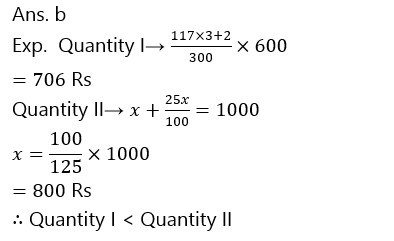(a) Quantity I >Quntity II

(b) Quantity I < Quantity II

(c) Quantity I ≤ Quantity II

(d)Quantity I=quantity II or No relation

(e) Quantity I ≥ Quantity II1. The largest possible right circular cylinder is cut out from a wooden cube of edge 7 cm.

Quantity I: volume of the cube left over after cutting out the cylinder

Quantity II: Total Surface area of cube remained after cutting out the cylinder.

Note: compare the magnitudes of both quantities.

(a) Quantity I > Quantity II

(b) Quantity I < Quantity II

(c) Quantity I ≥ Quantity II

(d) Quantity I ≤ Quantity II

(e) Quantity I = Quantity II or No relation1. Quantity I: difference between the largest and the smallest part of the sum. A sum of Rs.1440 is lent out in three parts in such a way that the simple interests on first part at 2% for 3 years, second part at 3% for 4 years and third part at 4% for 5 years are equal.

Quantity II: Rs.460

(a) Quantity I > Quantity II

(b) Quantity I < Quantity II

(c) Quantity I ≥ Quantity II

(d) Quantity I ≤ Quantity II

(e) Quantity I = Quantity II or No relationDirections (6-10): Study the following pie-chart and answer the questions that follow it.

Given below are the two pie charts which shows the percentage distribution of admission of students in five different schools in year 2016 and 2017.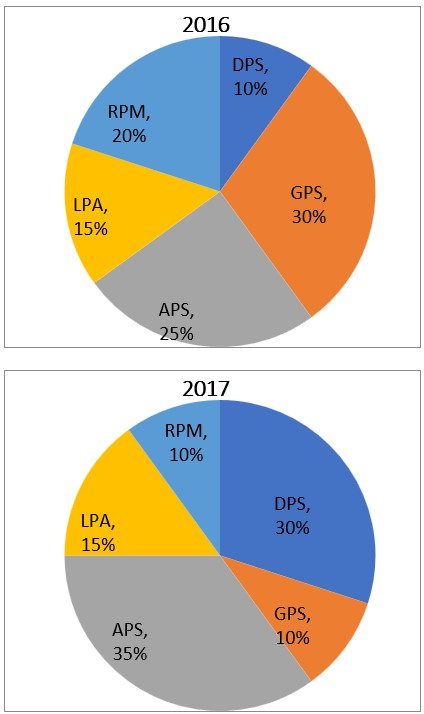1. Total number of admission in 2016 and 2017 are 2000 and 2500 respectively. Number of students in DPS in 2016 is what percent less or more than number of students in GPS in 2017?

(a) 35%

(b) 25%

(c) 40%

(d) 20%

(e) None of these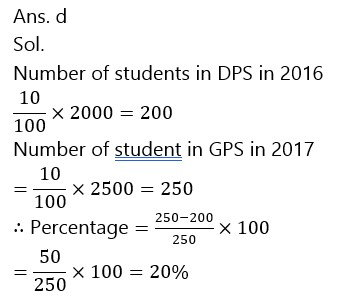1. If the total number of students in 2016 is 4000 and ratio of boys and girls in RPM is 2 : 3. Then the difference between boys and girls in APS is?

(a) 500

(b) 600

(c) Cannot be determined

(d) 400

(e) None of these

Ans. c

Sol.

Since ratio of number of boys and girls in 2016 in APS is not given.

1. If the total number of admission in 2017 is 5000 and 500 students left DPS in 2017 and taken admission in RPM in 2017 then number of admissions in RPM increases by what percent ?

(a) 80%

(b) 100%

(c) 120%

(d) 60%

(e) None of these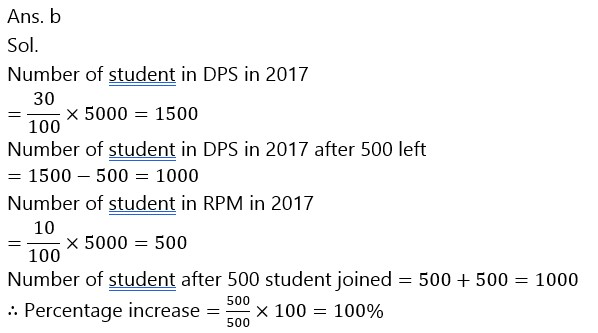1. If total student taking admission 2017 is 6000 and in 2016 is 4000. Then find the ratio of total student taking admission in RPM and LPA in 2016 and total student taking admission in GPS and LPA in 2017?

(a) 14 : 15

(b) 15 : 14

(c) 12 : 14

(d) 12 : 15

(e) None of these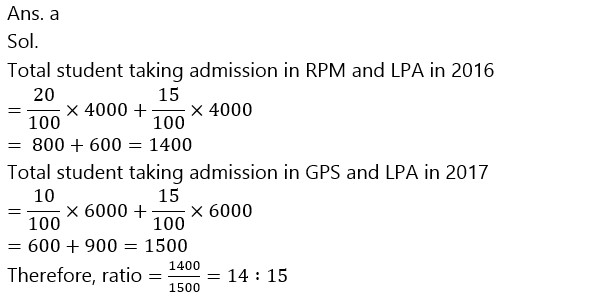1. If total student taking admission in 2016 and 2017 is 8000 and 10,000 respectively. And number of boys in GPS is 400 in 2016 and number of girls in DPS in 2017 is 1000. Then find the ratio of number of girls in GPS in 2016 to the number of boys in DPS in 2017 is ?

(a) 1 : 2

(b) 2 : 1

(c) 1 : 3

(d) 1 : 4

(e) 1 : 1